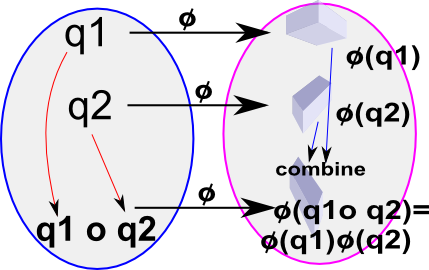# Maths - Morphism Examples

Here we look at some examples to see if they are morphisms, that is, does:

φ(v1) o φ(v2) = φ(v1 o v2)

where:

• φ = isomorphism mapping
• v1 and v2 = elements of group
• o = group operation## Example 1 vector addition with mapping = shift of originWith mapping φ between vector additions of fixed offset Vs so each vector is transformed by:

φ(V) = V + Vs

This is not an isomorphism since:

(V1 + Vs) + (V2 + Vs) ≠ (V1 + V2) + Vs

however:

(V1 + Vs) + (V2) = (V1 + V2) + Vs

so if we treat V2 as an offset and don't transform it then the equation matches.

## Example 2 vector addition with mapping = scalingWith mapping φ between vector spaces of scalar multiplication by M so each vector is transformed by:

φ(V) = M*V

This is an isomorphism since:

V1*M + V2*M = (V1 + V2) *M

## Example 3 multiplication with mapping = scalingWith mapping φ between vector additions of fixed scalar multiplication by M so each vector is transformed by:

φ(V) = M*V

This is not an isomorphism since:

V1*M * V2*M ≠ (V1 * V2) *M

So what mapping φ can we use with multipication to form an isomrphism? A common form is the 'sandwich product':

φ(v) = q v q-1

I have used q here to indicate a quaternion because the various types of vector product don't really have the correct properties. Use of the sandwich product in this way is discussed on this page.

so

 φ(q1*q2) = q q1 q2 q-1 = q q1 q-1 q q2 q-1 = φ(q1)*φ(q2) (since q-1 q =1)

So we can see that this sandwich product (conjugacy) has the right properties for an isomorphism

Where I can, I have put links to Amazon for books that are relevant to the subject, click on the appropriate country flag to get more details of the book or to buy it from them.Contemporary Abstract Algebra written by Joseph Gallian - This investigates algebra (Groups, Rings, Fields) using an abstract (set theory) approach. It is very readable for such an abstract topic but the inclusion of 1 page histories of the great mathematicians and other background information.